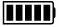ECE4253 Digital Communications Department of Electrical and Computer Engineering - University of New Brunswick, Fredericton, NB, Canada

Galois Field GF(2) Calculator

 Model C-172 POLYNOMIAL CALCULATORA:

B:

 A + B A - B A × B A / B

Discussion  Polynomials  MATLAB

1001110110
------------ = 1111110, Remainder = 000
1101

Detailed Calculation

 1111110 ------------ 1101 ) 1001110110 1101 ---- 100110110 1101 ---- 10010110 1101 ---- 1000110 1101 ---- 101110 1101 ---- 11010 1101 ---- 000

MATLAB Example

Calculations in GF(2) are performed by MATLAB functions found within the Communications Toolbox.

>> a = gf( [1 0 0 1 1 1 0 1 1 0] );

>> b = gf( [1 1 0 1] );

>> [q,r] = deconv(a,b) % deconvolution is equivalent to division

q = GF(2) array.

Array elements =

1      1      1      1      1      1      0

r = GF(2) array.

Array elements =

0

 2022-01-24 21:43:34 AST Last Updated: 2010-04-29 Richard Tervo [ tervo@unb.ca ]